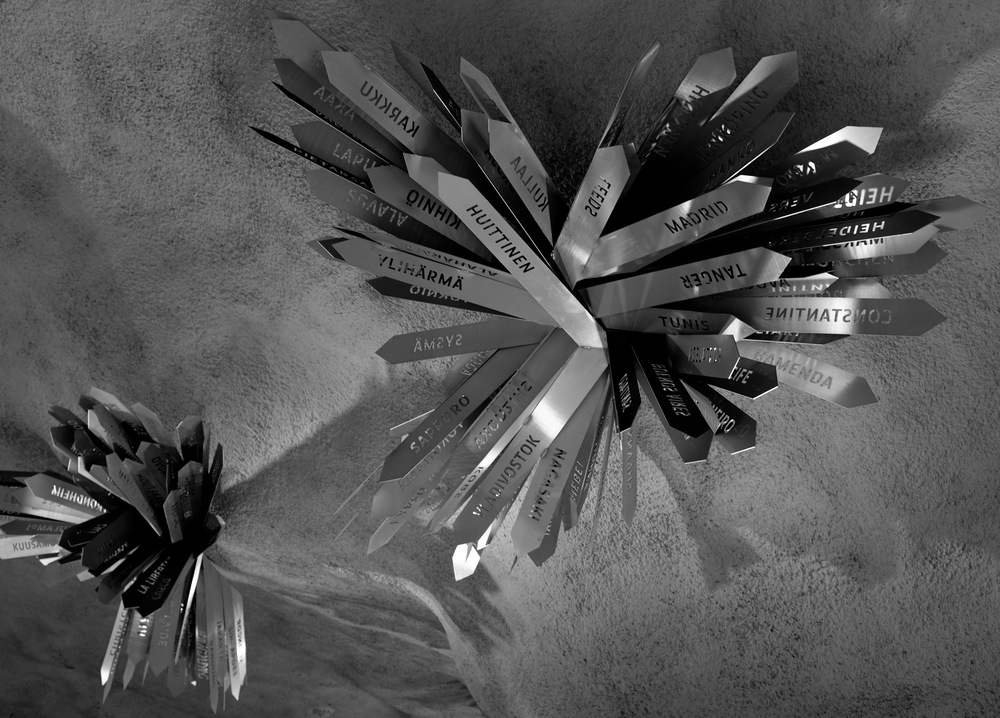# How I solved the Orbital Challenge

## 2016-05-17Reaktor, a local IT consulting company, recently posted a programming puzzle called Reaktor Orbital Challenge. You’re given a starting point and an end point on the globe and the locations for a bunch of communication satellites. The task is to find a route from the starting point to the end point via the satellites. Preferably the route should be the shortest possible one. The satellites and the ground stations can only communicate if they have a line-of-sight.

An Oculus Rift was drawn between the participants. This generated some buzz among the Finnish programming community. I especially enjoyed the low-effort solutions like copy-pasting the coordinates to Google Maps and eyeballing the route.

I have a bit of a love/hate relation with this kind of recruitment puzzles: I wouldn’t want to spend time on them, but they nerd-snipe me so easily. Therefore I decided to aim for a low-effort solution that still finds the shortest path. Let me tell you about it.

I wrote my solution in Clojure and used the graph library Loom, the linear algebra library core.matrix and the utility library potpuri. You can find the full source here.

## The idea

Finding the shortest route is an instance of the well-known problem of finding the shortest path between two nodes in a weighted graph. Satellites are the nodes and they’re connected if they can see each other. The weight of an edge is the distance between satellites. The ground stations need to be included as well.

There are so few satellites that you do not need to do anything clever - using a standard algorithm would be fine performance-wise. I looked up graph libraries for Clojure and decided to go with Loom, which has an implementation of Dijkstra’s algorithm.

The locations are given in longitude/latitude/altitude coordinates. I realized that you could do the calculations in this coordinate system, but I didn’t know how to do it and I didn’t know if it would be easy (turns out it is). Converting to the Euclidean coordinates is easy and I knew how to work with them, so that’s what I did.

How do you build the graph, then? Calculating the weight is easy, we can just use the Euclidean distance of the points. All that is left is to check that the points can see each other. As we will see below, it’s not very hard either.

## Coordinate transform

Initially I did the coordinate transform the same way as I’ve done it since I was 15 years old: by looking up rotation matrices and multiplying them. Later I realized I should’ve looked up this thing instead. The problem statement says that Earth is a perfect sphere with the radius of 6371 km. Thus in Wikipedia’s formulas, $N(\phi) = 6371\ \textrm{km}$ and $e = 0$. In Clojure:

(def earth-radius 6371)

(defn geo->xyz [[lat lon alt]]
"Convert latitude/longitude/altitude to Euclidean coordinates."
[(* h (Math/cos lat) (Math/cos lon))
(* h (Math/cos lat) (Math/sin lon))
(* h (Math/sin lat))]))


I chose to represent x-y-z vectors as three-element Clojure vectors. This is both concise and understood by core.matrix.

When doing this conversion, you have to fix the origin and the directions for the Euclidean axes. I did it the “obvious” way:

1. We use right-handed coordinate system.
2. The origin is in the center of the Earth.
3. The positive Z axis goes through the North Pole.
4. The positive X axis goes through the prime meridian.

When looking the formulas up, I learned that this way has a name. It’s called Earth-Centered, Earth-Fixed coordinate system.

## Line of sight

Next, we have two satellites and the Earth. How do we know if the satellites can see each other and that the Earth is not between them?

One way is to write the equations for the sphere of Earth and the line segment between the satellites and to solve for the intersection points. Another way is to calculate the distance between the line segment and the center of Earth. If the distance is less than Earth’s radius, there’s an intersection.

I always think about the distance between a line and a point as projecting the point to the line. It was quick to write the required calculations and I even got them right on the first try! The vector operations are kindly provided by core.matrix.

(defn point-line-segment-dist
"Compute the shortest distance between the line segment a-b and point c."
[a b c]
(let [k (- b a)
l (- c a)
;; scalar projection of l onto k.
t (/ (dot l k) (norm k))]
(distance (+ a (* (clamp t 0 1) k)) c)))

(defn line-of-sight? [a b]
(<= earth-radius (point-line-segment-dist a b [0 0 0])))


## Building the graph

The input file looks something like this:

#SEED: 0.24904920277185738
SAT0,78.47003444920836,-80.16227317274806,556.3585069486544
SAT1,18.2619020748309,89.92023247596006,367.93737770788107
# ...lines omitted...
SAT19,43.337390738091216,117.63969735544856,541.2530580475883
ROUTE,-44.35263069870813,162.23137604080517,62.2777848501151,-90.67241530334475


I’m not going to bore you with the parsing code. I parsed the input into a map like this:

{"SAT0" [78.47003444920836 -80.16227317274806 556.3585069486544]
;; ...lines omitted...
:start [-44.35263069870813 162.23137604080517 0]
:end [62.2777848501151 -90.67241530334475 0]}


Now all that is needed is a sequence of edges and weights for Loom:

(defn build-graph [data]
(let [xyz-data (map-vals geo->xyz data)]
(apply weighted-graph
(for [[key1 pos1] xyz-data
[key2 pos2] xyz-data
:when (and (not= key1 key2)
(not= #{:start :end} (set [key1 key2]))
(line-of-sight? pos1 pos2))]
[key1 key2 (distance pos1 pos2)]))))


Our work here is done.

(defn get-route [graph]
(butlast (rest (dijkstra-path graph :start :end))))


For the full solution, see my gist. It took me 80 lines of Clojure and about an hour, so the effort was sufficiently low.